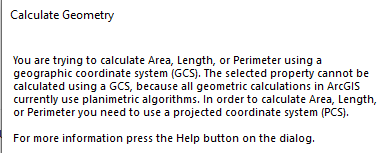# Shape_Length and Shape_Area of Geographic Coordinate Data

344
1
06-13-2019 05:20 PM
Highlighted
Occasional Contributor III

Suppose you have a spatial data expressed in a  geographic coordinate system (eg WGS84).

Many geoprocessing tools, when operating on datasets, add one or  two fields to the data: Shape_Length (for lines and polygons), and Shape_Area (for polygons). For instance, suppose you select certain polygons by pointing and clicking, and then request a new dataset be made from the selections -- you will get a new dataset with these two fields added (if they did not already exist). And the values in these fields will be measured in decimal degrees, or squared decimal degrees (I guess the latter should be called stedegrees, by analogy with steradians).

Now, if you take your same data, create a new column, click on that column, choose calculate geometry, and choose Use coordinate system of data source you will get an error. The message is to the effect that arcmap has no algorithm with which to make such a calculation (see attachment below).So how then, or more importantly why, is that field being populated by other geoprocessing tools. Presumably the numbers are incorrect since arcmap says they have no algorithm to calculate them. A possible algorithm would, of course, be calculate the linear distance and then convert that to an angle by assuming it was a great circle arc on the earth's surface.

This is just one of many inconsistencies in the software.

by